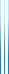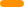# PublicationsPapers

## Inequalities on submodular functions via term rewriting

Yuval Filmus
Information Processing Letters, Volume 113, Issue 13, 2013, pp. 457–464

We devise a method for proving inequalities on submodular functions, with a term rewriting flavor. Our method comprises of the following steps:

• Start with a linear combination $X$ of the values of the function.
• Define a set of simplification rules.
• Conclude that $X \ge Y$, where $Y$ is a linear combination of a small number of terms which cannot be simplified further.
• Calculate the coefficients of $Y$ by evaluating $X$ and $Y$ on functions on which the inequality is tight.

The crucial third step is non-constructive, since it uses compactness of the dual cone of submodular functions. Its proof uses the classical uncrossing technique with a quadratic potential function.

We prove several inequalities using our method, and use them to tightly analyze the performance of two natural (but non-optimal) algorithms for submodular maximization, the random set algorithm and local search.

## BibTeX

@article{Filmus2013,
author = {Yuval Filmus},
title = {Inequalities on submodular functions via term rewriting},
journal = {Information Processing Letters},
volume = {113},
issue = {13},
year = {2013},
pages = {457--464}
}copy to clipboard